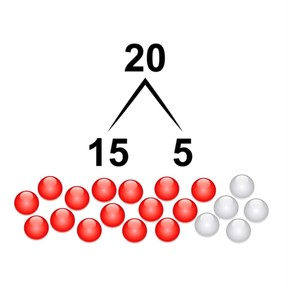Decomposing and composing numbers to 20

# Decomposing and composing numbers to 20

Decomposing and composing numbers to 20

No account needed.8,000 schools use Gynzy92,000 teachers use Gynzy1,600,000 students use Gynzy

## General

Students learn to decompose and compose numbers to 20. They learn to recognize decomposition in images, but also using number bond arrows and numbers. They learn to compose numbers by counting on.

## Relevance

It is useful to know different ways of splitting an amount. You can split 15 cookies, or other objects into groups of 5 and 10, but also 6 and 9. Ask students to come up with other number bonds.

## Introduction

Students practice counting amounts to 20 by counting vegetables.

## Development

Show that you can decompose numbers in different ways. Use the fish to demonstrate decomposing the number 12. Show that you can turn that into 6 and 6, but also 9 and 3. This is called decomposing a number. If you already have one of the numbers, you simply count on to see how many are needed. Explain that that is called composing a number. Show decomposition by dividing the 14 fish between two fishbowls. Ask students to write the number bonds down. Next show a few decompositions of 7. Ask students what the decomposition is if you switch the numbers, and discuss all the possible decompositions of 7. Next, explain that it is sometimes possible to see the decomposition in an image by color and that the numbers in a number bond can be switched. Discuss that you can also use an arrow to show the number bonds. Practice recognizing the numbers in the images with beads and discuss with students which numbers they can switch, and where number bonds and arrows could be used. Explain that when a decomposition isn't visible by color, that you count on to discover the missing number. Practice this together and check that students are able to count on. There are also decompositions that only have a number. Ask the students to decompose in their heads, but they can use blocks to support their thinking.

Check that students understand decomposing and composing numbers to 20 by asking the following questions:
- What does it mean to decompose a number? And composing a number?
- How can you check if your number bond is correct? (count on)
- Can you name two decompositions of 16?

## Guided Practice

Students practice decompositions by first filling in a missing number with visual support and then are asked to decompose in their heads, without visual support.

## Closing

Check that students can decompose and compose numbers to 20 by asking them to fill in the missing number from the number bond. Discuss that it is useful to be able to decompose numbers so you know the different ways that you can divide something. Ask students to come up with other examples where you can use decomposing or composing.

## Teaching Tip

Students who are challenged by this goal can be supported by the use of manipulatives while decomposing and composing numbers.

## Instruction materials

Blocks

### The online teaching platform for interactive whiteboards and displays in schools

• Save time building lessons

• Manage the classroom more efficiently

• Increase student engagement